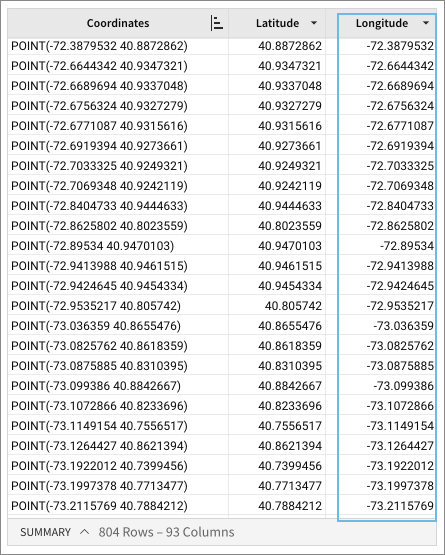# Longitude

The Longitude function obtains the longitude of a point.

The input column must be a valid location within the Geographic Coordinate System.

You can use the Longitude function on Snowflake and BigQuery connections.

Longitude is one of Sigma's Geography functions.

## Syntax

`Longitude(point)`

The function argument is:

point
Required
A valid point value that can be located on the Earth's sphere; must comply with the Geographic Coordinate System
The function does not support other coordinate types, such as lines, polygons, and so on.

## Example

`Longitude([Coordinates])`

The function Longitude extracts the latitude of a point from the Coordinate column: